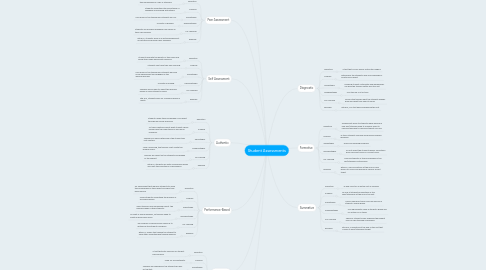# Student Assessments

Get Started. It's FreeStudent Assessments## 1. Diagnostic

### 1.1. Definition

1.1.1. A test that occurs before instruction begins.

### 1.2. Purpose

1.2.1. Determines the student's level of knowledge of a particular subject.

1.3.1. Knowing student's strengths and weaknesses can help the teacher better plan the unit.

1.4.1. Can take up a lot of time.

### 1.5. For Learning

1.5.1. Informs the teacher what the students already know and what they need to know.

### 1.6. Example

1.6.1. 8th ELA, Pre-test before beginning the unit.

## 2. Formative

### 2.1. Definition

2.1.1. Assessment given to students while learning a new skill/standard used to measure ways to improve teaching to improve student success.

### 2.2. Purpose

2.2.1. To track students learning and provide ongoing feedback.

2.3.1. Quick and ongoing feedback.

2.4.1. Do not affect the students grade. Formatives have low point value or no point value.

### 2.5. For Learning

2.5.1. Informs students of their knowledge of the skill/standard continuously.

### 2.6. Example

2.6.1. 8th ELA, daily formatives at the end of each lesson on nouns and pronouns used as an exit ticket.

## 3. Summative

### 3.1. Definition

3.1.1. To end result of an entire unit of learning.

### 3.2. Purpose

3.2.1. To look at students understand of the skills/standards at the end of the unit.

3.3.1. Have a high point value and can improve a student's overall grade.

3.4.1. Can significantly lower a student's grade and no option for re-takes.

### 3.5. For Learning

3.5.1. Shows if student's have achieved the highest level of rigor through scaffolding.

### 3.6. Example

3.6.1. 8th ELA, summative at the end of the unit that covers all skills/standards taught.

## 4. Performance-Based

### 4.1. Definition

4.1.1. An assessment that requires students to show their knowledge on the subject and skills they have learned.

### 4.2. Purpose

4.2.1. Helps students understand the process of solving problems.

4.3.1. Gives teaches more knowledge about  the learning needs of their students.

4.4.1. No right or wrong answers, so teaches need to create a grade-able rubric.

### 4.5. For Learning

4.5.1. The purpose of performance-based is to determine the student's progress

### 4.6. Example

4.6.1. 8th ELA, essays that require the student to show their understanding through analysis.

## 5. High Stakes

### 5.1. Definition

5.1.1. A test that puts pressure on student performance.

### 5.2. Purpose

5.2.1. Used for accountability.

5.3.1. Teachers are rewarded if the students do well on the test.

5.4.1. Teachers are sanctioned if the students do not do well on the test.

### 5.5. Of Learning

5.5.1. Measures the students performance and compares to other schools in the district.

### 5.6. Example

5.6.1. 8th ELA, TNReady state standardized tests.

## 6. Portfolio

### 6.1. Definition

6.1.1. An evaluation tool measured through a collection of student work.

### 6.2. Purpose

6.2.1. To show student's achievements throughout the school year.

6.3.1. Student-centered learning. Can create higher levels of self evaluation for students.

6.4.1. Can consume a lot of time to plan and grade the entire portfolio.

### 6.5. Of Learning

6.5.1. Measures student's success and records it in one place, the portfolio.

### 6.6. Example

6.6.1. 8th ELA, student's will keep all of their writing assignments in a folder throughout the year. At the end of the year students will be graded on their improvements in writing.

## 7. Authentic

### 7.1. Definition

7.1.1. Student's apply their knowledge of a subject through real-world problems.

### 7.2. Purpose

7.2.1. To think creatively about what student's have learned and the importance of real-world problems.

7.3.1. Teacher can freely determine if the student has met mastery.

7.4.1. Time consuming, the teacher must create the grading rubric.

### 7.5. Of Learning

7.5.1. Teacher will report on the student's knowledge of the subject.

### 7.6. Example

7.6.1. 8th ELA, students will write a persuasive essay and learn the importance of persuasion.

## 8. Self Assessment

### 8.1. Definition

8.1.1. Students evaluate the quality of their work and grade then make appropriate revisions.

### 8.2. Purpose

8.2.1. Students can track their own learning.

8.3.1. Less work for the teacher and students become more independent and engaged in the learning process.

### 8.5. For Learning

8.5.1. Teachers will be able to adjust the learning based on each student's needs.

### 8.6. Example

8.6.1. 8th ELA, students work on a research paper in drafts.

## 9. Peer Assessment

### 9.1. Definition

9.1.1. Students collaborate with peers on a task of their knowledge of a skill or standard.

### 9.2. Purpose

9.2.1. Students understand the importance of feedback and working with others.

9.3.1. Less work for the teacher and students are coll

### 9.5. For Learning

9.5.1. Students are provided feedback from peers on their own learning.

### 9.6. Example

9.6.1. 8th ELA, students work on a writing assignment in partners and provide daily feedback.